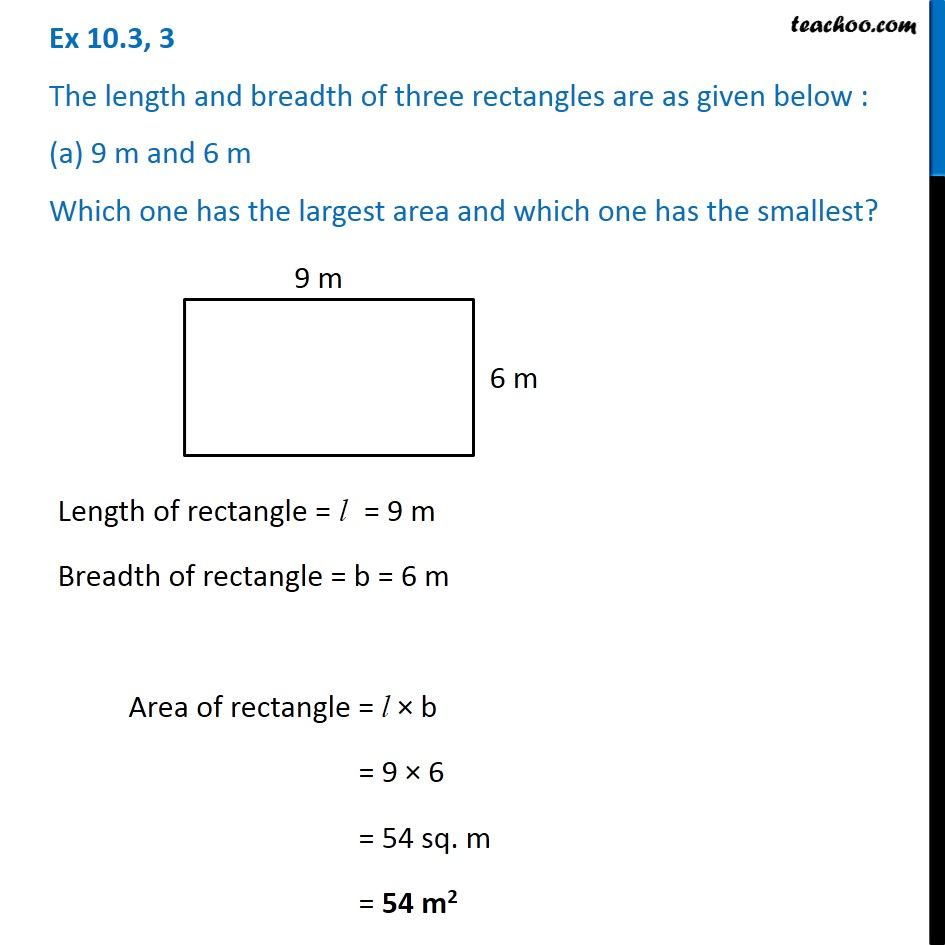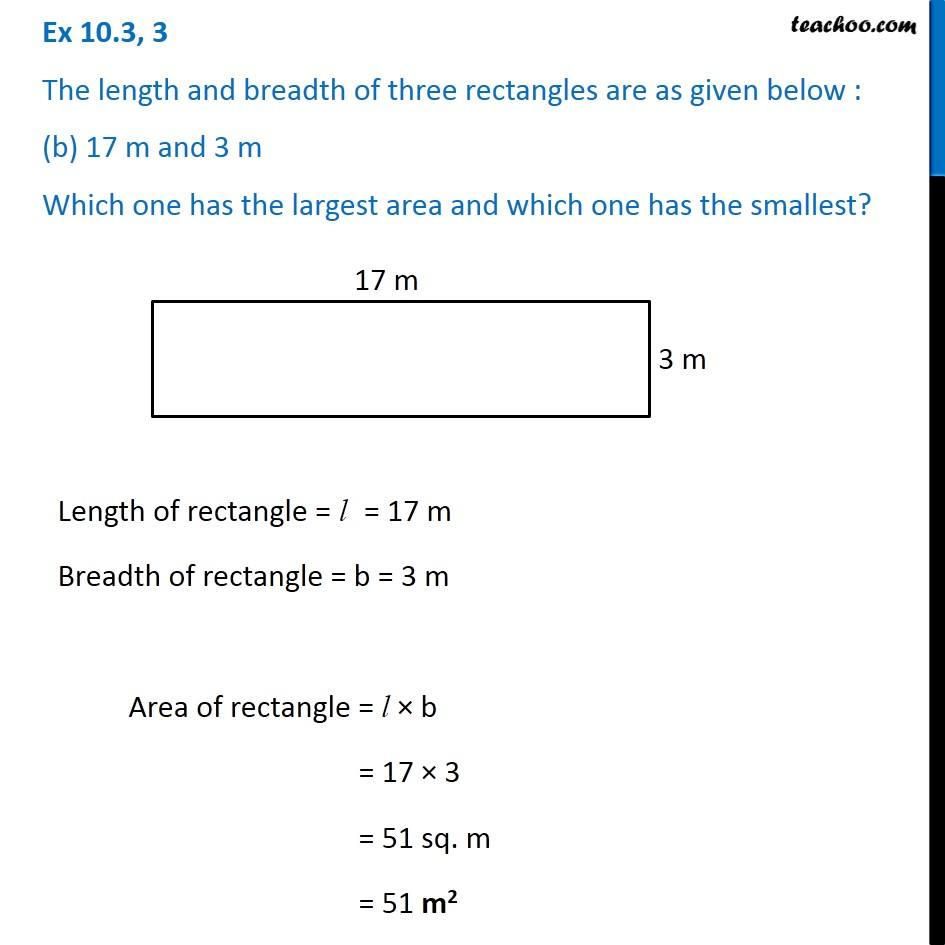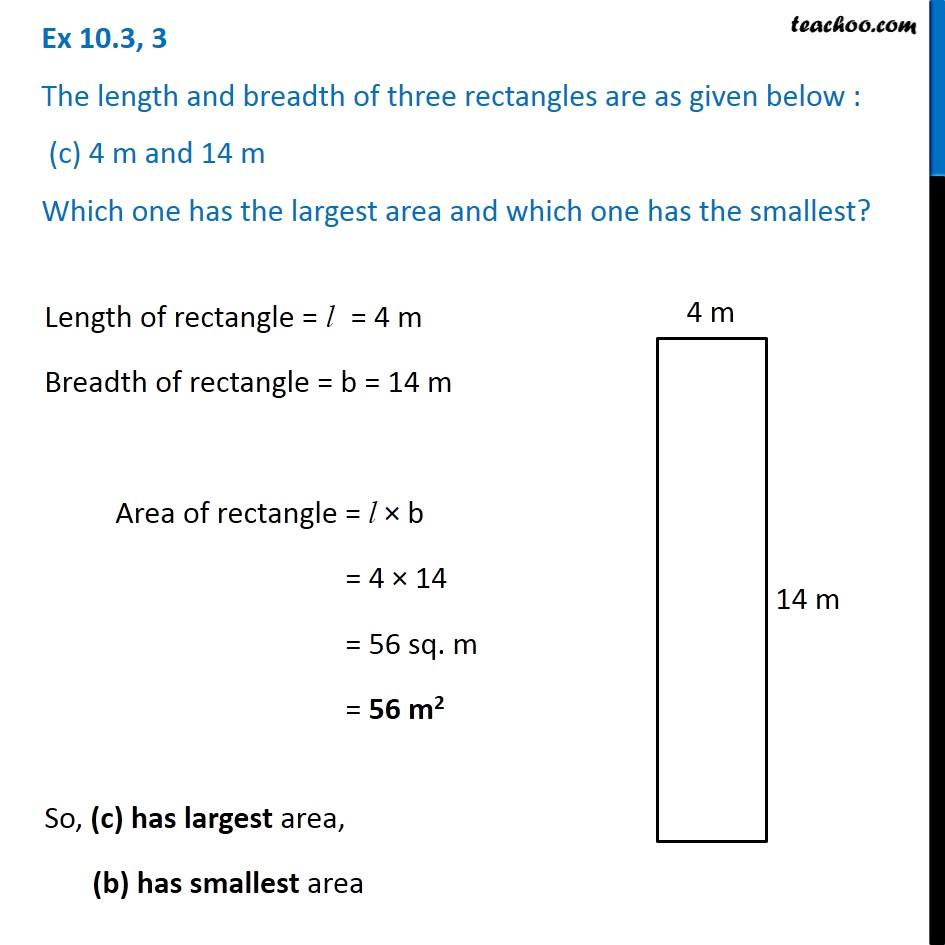Subscribe to our Youtube Channel - https://you.tube/teachoo

1. Chapter 10 Class 6 Mensuration
2. Concept wise
3. Area of Rectangle

Transcript

Ex 10.3, 3 The length and breadth of three rectangles are as given below : (a) 9 m and 6 m Which one has the largest area and which one has the smallest? Length of rectangle = l = 9 m Breadth of rectangle = b = 6 m Area of rectangle = l × b = 9 × 6 = 54 sq. m = 54 m2 Ex 10.3, 3 The length and breadth of three rectangles are as given below : (b) 17 m and 3 m Which one has the largest area and which one has the smallest? Length of rectangle = l = 17 m Breadth of rectangle = b = 3 m Area of rectangle = l × b = 17 × 3 = 51 sq. m = 51 m2 Ex 10.3, 3 The length and breadth of three rectangles are as given below : (c) 4 m and 14 m Which one has the largest area and which one has the smallest? Length of rectangle = l = 4 m Breadth of rectangle = b = 14 m Area of rectangle = l × b = 4 × 14 = 56 sq. m = 56 m2 So, (c) has largest area, (b) has smallest area

Area of Rectangle